# Monetary Policy Model¶

A central bank must set nominal interest rate so as to minimize deviations of inflation rate and GDP gap from established targets.

A monetary authority wishes to control the nominal interest rate $x$ in order to minimize the variation of the inflation rate $s_1$ and the gross domestic product (GDP) gap $s_2$ around specified targets $s^∗_1$ and $s^∗_2$, respectively. Specifically, the authority wishes to minimize expected discounted stream of weighted squared deviations

\begin{equation} L(s) = \frac{1}{2}(s − s^∗)'\Omega(s − s^∗) \end{equation}

where $s$ is a $2\times 1$ vector containing the inflation rate and the GDP gap, $s^∗$ is a $2\times 1$ vector of targets, and $\Omega$ is a $2 \times 2$ constant positive definite matrix of preference weights. The inflation rate and the GDP gap are a joint controlled exogenous linear Markov process

\begin{equation} s_{t+1} = \alpha + \beta s_t + \gamma x_t + \epsilon_{t+1} \end{equation}

where $\alpha$ and $\gamma$ are $2 \times 1$ constant vectors, $\beta$ is a $2 \times 2$ constant matrix, and $\epsilon$ is a $2 \times 1$ random vector with mean zero. For institutional reasons, the nominal interest rate $x$ cannot be negative. What monetary policy minimizes the sum of current and expected future losses?

This is an infinite horizon, stochastic model with time $t$ measured in years. The state vector $s \in \mathbb{R}^2$ contains the inflation rate and the GDP gap. The action variable $x \in [0,\infty)$ is the nominal interest rate. The state transition function is $g(s, x, \epsilon) = \alpha + \beta s + \gamma x + \epsilon$

In order to formulate this problem as a maximization problem, one posits a reward function that equals the negative of the loss function $f(s,x) = −L(s)$

The sum of current and expected future rewards satisfies the Bellman equation

\begin{equation} V(s) = \max_{0\leq x}\left\{-L(s) + \delta + E_\epsilon V\left(g(s,x,\epsilon)\right)\right\} \end{equation}

Given the structure of the model, one cannot preclude the possibility that the nonnegativity constraint on the optimal nominal interest rate will be binding in certain states. Accordingly, the shadow-price function $\lambda(s)$ is characterized by the Euler conditions

\begin{align} \delta\gamma'E_\epsilon \lambda\left(g(s,x,\epsilon)\right) &= \mu \\ \lambda(s) &= -\Omega(s-s^*) + \delta\beta'E_\epsilon \lambda\left(g(s,x,\epsilon)\right) \end{align}

where the nominal interest rate $x$ and the long-run marginal reward $\mu$ from increasing the nominal interest rate must satisfy the complementarity condition \begin{equation} x \geq 0, \qquad \mu \leq 0, \qquad x > 0 \Rightarrow \mu = 0 \end{equation}

It follows that along the optimal path

\begin{align} \delta\gamma'E_\epsilon \lambda_{t+1} &= \mu_t \\ \lambda_t &= -\Omega(s_t-s^*) + \delta\beta'E_\epsilon \lambda_{t+1}\\ x \geq 0, \qquad \mu \leq 0, &\qquad x > 0 \Rightarrow \mu = 0 \end{align}

Thus, in any period, the nominal interest rate is reduced until either the long-run marginal

reward or the nominal interest rate is driven to zero.

In :
import numpy as np
import matplotlib.pyplot as plt
from mpl_toolkits.mplot3d import Axes3D
from matplotlib import cm
from compecon import BasisChebyshev, DPmodel, BasisSpline, qnwnorm, demo
import pandas as pd
pd.set_option('display.float_format',lambda x: f'{x:.3f}')


### Model Parameters¶

In :
α   = np.array([[0.9, -0.1]]).T             # transition function constant coefficients
β    = np.array([[-0.5, 0.2], [0.3, -0.4]])  # transition function state coefficients
γ   = np.array([[-0.1, 0.0]]).T             # transition function action coefficients
Ω   = np.identity(2)                        # central banker's preference weights
ξ   = np.array([[1, 0]]).T                  # equilibrium targets
μ   = np.zeros(2)                           # shock mean
σ   = 0.08 * np.identity(2),                # shock covariance matrix
δ   = 0.9                                   # discount factor


### State Space¶

There are two state variables: 'GDP gap' = $s_0\in[-2,2]$ and 'inflation'=$s_1\in[-3,3]$.

In :
n = 21
smin = [-2, -3]
smax = [ 2,  3]

basis = BasisChebyshev(n, smin, smax, method='complete',
labels=['GDP gap', 'inflation'])


### Action space¶

There is only one action variable x: the nominal interest rate, which must be nonnegative.

In :
def bounds(s, i, j):
lb  = np.zeros_like(s)
ub  = np.full(lb.shape, np.inf)
return lb, ub


### Reward Function¶

In :
def reward(s, x, i, j):
s = s - ξ
f = np.zeros_like(s)
for ii in range(2):
for jj in range(2):
f -= 0.5 * Ω[ii, jj] * s[ii] * s[jj]
fx = np.zeros_like(x)
fxx = np.zeros_like(x)
return f, fx, fxx


### State Transition Function¶

In :
def transition(s, x, i, j, in_, e):
g = α + β @ s + γ @ x + e
gx = np.tile(γ, (1, x.size))
gxx = np.zeros_like(s)
return g, gx, gxx


The continuous shock must be discretized. Here we use Gauss-Legendre quadrature to obtain nodes and weights defining a discrete distribution that matches the first 6 moments of the Normal distribution (this is achieved with m=3 nodes and weights) for each of the state variables.

In :
m   = [3, 3]
[e,w] = qnwnorm(m,μ,σ)


### Model structure¶

In :
bank = DPmodel(basis, reward, transition, bounds,
x=['interest'], discount=δ, e=e, w=w)


In :
bank_lq = bank.lqapprox(ξ,0)



If Nonnegativity Constraint Violated, Re-Compute Deterministic Steady-State

In :
if xstar < 0:
I = np.identity(2)
xstar = 0.0
sstar = np.linalg.solve(np.identity(2) - β, α)

frmt = '\t%-21s = %5.2f'
print(frmt % ('GDP Gap', sstar))
print(frmt % ('Inflation Rate', sstar))
print(frmt % ('Nominal Interest Rate', xstar))

Deterministic Steady-State
GDP Gap               =  0.61
Inflation Rate        =  0.06
Nominal Interest Rate =  0.00


### Solve the model¶

We solve the model by calling the solve method in bank. On return, sol is a pandas dataframe with columns GDP gap, inflation, value, interest, and resid. We set a refined grid nr=5 for this output.

In :
S = bank.solve(nr=5)

Solving infinite-horizon model collocation equation by Newton's method
iter change       time
------------------------------
0       6.7e+00    0.3994
1       9.9e-01    1.1321
2       9.1e-04    1.7787
3       8.3e-07    2.2782
4       7.7e-09    2.7687
Elapsed Time =    2.77 Seconds


To make the 3D plots, we need to reshape the columns of sol.

In :
S3d = {x: S[x].reshape((5*n,5*n)) for x in S.columns}


This function will make all plots

In :
def makeplot(series,zlabel,zticks,title):
fig = plt.figure(figsize=[8,5])
ax = fig.gca(projection='3d')
ax.plot_surface(S3d['GDP gap'], S3d['inflation'], S3d[series], cmap=cm.coolwarm)
ax.set_xlabel('GDP gap')
ax.set_ylabel('Inflation')
ax.set_zlabel(zlabel)
ax.set_xticks(np.arange(-2,3))
ax.set_yticks(np.arange(-3,4))
ax.set_zticks(zticks)
ax.set_title(title)


### Optimal policy¶

In :
fig1 = makeplot('interest', 'Nomianal Interest Rate',
np.arange(0,21,5),'Optimal Monetary Policy')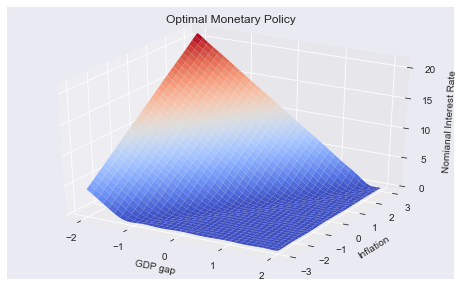### Value function¶

In :
fig2 = makeplot('value','Value',
np.arange(-12,S['value'].max(),4),'Value Function')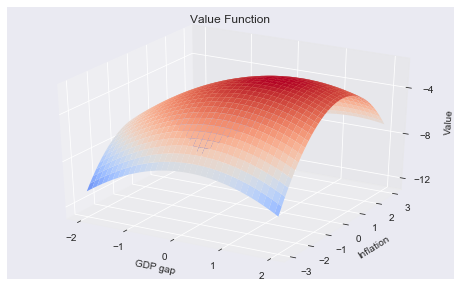### Residuals¶

In :
fig3 = makeplot('resid','Residual',
[-1.5e-3, 0, 1.5e3],'Bellman Equation Residual')
plt.ticklabel_format(style='sci', axis='z', scilimits=(-1,1))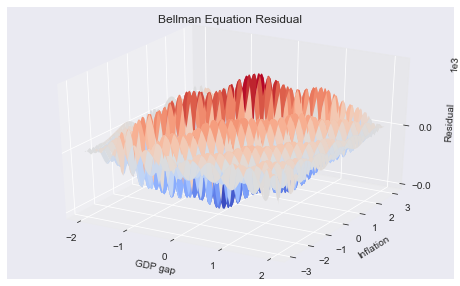## Simulating the model¶

We simulate 21 periods of the model starting from $s=s_{\min}$, 10000 repetitions.

In :
T = 21
nrep = 10_000
data = bank.simulate(T, np.tile(np.atleast_2d(smax).T,nrep))

In :
subdata = data[data['time']==T][['GDP gap', 'inflation', 'interest']]
'Ergodic Means': subdata.mean(),
'Ergodic Standard Deviations': subdata.std()})
stats.T

Out:
GDP gap inflation interest
Ergodic Means 0.591 0.058 0.301
Ergodic Standard Deviations 0.315 0.334 0.694

### Simulated State and Policy Paths¶

In :
subdata = data[data['_rep'].isin(range(3))]
opts = dict(spec='r*', offset=(0, -15), fs=11, ha='right')
gdpstar, infstar, intstar = stats['Ergodic Means']

fig = demo.figure('Simulated and Expected ' + ylabel,'Period', ylabel,[0, T + 0.5])
plt.plot(data[['time',series]].groupby('time').mean())
plt.plot(subdata.pivot('time','_rep',series),lw=1)
plt.xticks(np.arange(0,24,4))
plt.yticks(yticks)
return fig

In :
fig4 = simplot('GDP gap','GDP gap',np.arange(smin,smax+1),gdpstar)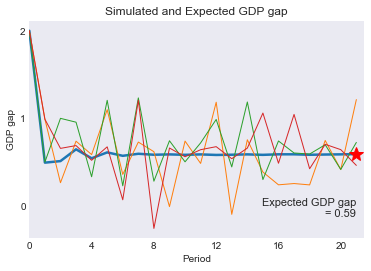In :
fig5 = simplot('inflation', 'Inflation Rate',np.arange(smin,smax+1),infstar)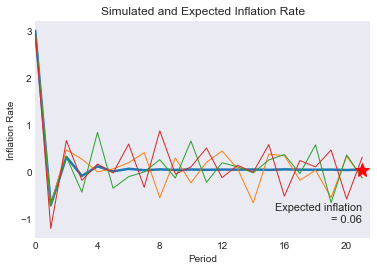In :
fig6 = simplot('interest','Nominal Interest Rate',np.arange(-2,5),intstar)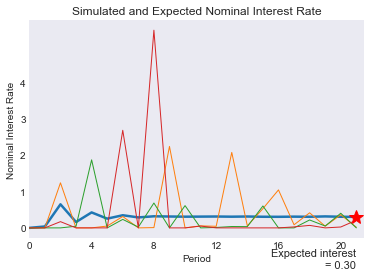In :
#demo.savefig([fig1,fig2,fig3,fig4,fig5,fig6])